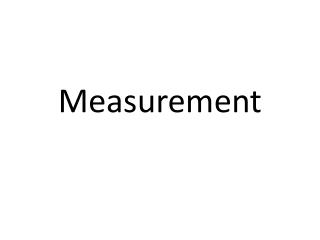Download PresentationMeasurement

# Measurement

Download Presentation## Measurement

- - - - - - - - - - - - - - - - - - - - - - - - - - - E N D - - - - - - - - - - - - - - - - - - - - - - - - - - -
##### Presentation Transcript

1. Measurement

2. Measurement • Measurements have 2 parts: • A number • A unit • Example: 5cm • Scientists (and we will) use the SI system • Système International d’unités

3. The SI units = METRIC • There are only 3 countries in the whole world using the ENGLISH SYSTEM of measurement • Liberia • Myanmar (formerly Burma) • THE UNITED STATES of AMERICA • Why is the metric system so much easier? • Most countries use it • It is based on powers of 10

4. Units • There is always a BASE unit for every measurement • There can be prefixes added to the base to make it larger or smaller Example: millisecond, kilogram

5. Base Units • Length = meter (m) • Mass = gram (g) • Volume = liter (L) or cubic centimeters (cm3) • Time = second (s) ** Prefixes multiply the BASE unit by a value of 10 **

6. PREFIX Symbol multiplier Kilo k 1,000 hecto h 100 dekada 10 base: 1 deci d 0.1 centi c 0.01 milli m 0.001

7. King Henry Died Unfortunately Drinking Chocolate Milk

8. Unit Conversions • The metric system is so useful because we can convert from one unit to another EASILY • Write out the abbreviations in a horizontal row: K H D U D C M • Find the location of the original unit, then count the number of jumps and direction to the new unit • Re-write the number, then hold the pencil on the decimal point. • Move your pencil, making “egg crates”, the number of jumps in the correct direction- then fill in the empy crates with ZEROS • Re-write your final answer, including number and unit

9. Let’s try some! Convert: 12 gram to kilograms 87.25 hL to cL 5.22 seconds to milliseconds 99.99 dm to cm How many centiliters are in 1 kiloliter?

10. Length • Length = distance from one point to another • Length is measured with a METRIC RULER • The BASE unit = meter • Examples: centimeter (cm), millimeter (mm), decimeter (dm)

11. Mass • Mass= the amount of matter in an object • Mass is measured with a balance • The BASE unit = gram • Examples: kilogram (kg), milligram (mg)

12. Volume Volume= the amount of space something takes up SolidMeasurement Liquid Measurement The BASE unit = Liter Use a GRADUATED CYLINDER to measure • The BASE unit = cm3 • Use a formula to calculate for regular shapes • For irregular shapes use Water Displacement Method

13. Volume Direct Measurement Indirect Measurement Water displacement method Fill a graduated cylinder with water- record volume Carefully add object- record new volume of water Subtract old volume from newvolume = volume of object • Use a ruler to measure and plug into formula • Example: Volume of a cube = length x width x height

14. In order for a measurement to be reliable… It needs to be both ACCURATE and PRECISE • Accuracy = EXACT • Precision = multiple measurements close to each other

15. Scientific Notation • Makes using large numbers easier • Write a number times a power of 10 • Example: • 3.0 x 108 = 300,000,000 • Number must be between 1 and 10 • Always there • The NUMBER of places and DIRECTION the decimal is moved

16. Scientific Notation • #’s less than 1 (0.30400823) have a NEGATIVE exponent • #’s greater than 1 have a POSITIVE exponent

17. Try Some: • Expand: • 4.2 x 104 • 3.359 x 10 -6 • 7.8112 x 108

18. Try Some More… • Write in scientific notation: • 870 • 0.0000789 • 0.245 • 75000